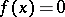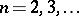Bolzano-Weierstrass selection principle

(diff) ← Older revision | Latest revision (diff) | Newer revision → (diff)
Depending on the criterion according to which the segments are chosen in applying the Bolzano–Weierstrass selection principle, the process obtained is effective or ineffective. An example of the former case is the application of the principle to prove that for a continuous real function that assumes values of opposite sign at the ends of a given segment, this segment contains a point at which the function vanishes (cf. Cauchy theorem on intermediate values of continuous functions). In this case the criterion chosen for the successive choice of the segments is that the function assumes values of different sign at the two ends of the chosen segment. If there is a way of computing the value of the function at every point, then, after performing a sufficient number of steps, it is possible to obtain the coordinates of the point at which the function vanishes, to within a given degree of accuracy. Thus, in addition to proving that a root of the equationexists on a segment at the ends of which the values of the function are of different sign, one also has a method of approximately solving this equation. An example of an ineffective process is the use of the Bolzano–Weierstrass selection principle to prove that a continuous real function on a segment attains a maximum on the segment. Here, the segment chosen in the successive subdivisions is the one on which the maximum of the values of the function is not less than that on the other one. If, as in the former case, it is possible to calculate the value of the function at any point, this is still not sufficient for an effective choice of the required segment. Accordingly, the Bolzano–Weierstrass selection principle can be used in this case only to prove an existence theorem which says that the function assumes its maximum at some point, but not to specify this point within a given degree of accuracy.
There exist various generalizations of the Bolzano–Weierstrass selection principle, e.g. to apply it in the-dimensional Euclidean space () to-dimensional cubes, which are successively subdivided into congruent cubes with side-lengths of one-half that of the original cube.